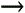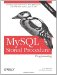# Operators

MySQL operators include the familiar operators common to most programming languages, although C-style operators (++,,+=, etc.) are not supported.

Operators are typically used within the SET statement to change the value of a variable, within comparison statements such as IF or CASE, and in loop control expressions. Example 3-10 shows a few simple examples of using operators within stored programs.

Example 3-10. Examples of operators in a stored program

 ```create procedure operators( ) begin DECLARE a int default 2; declare b int default 3; declare c FLOAT; set c=a+b; select 'a+b=',c; SET c=a/b; select 'a/b=',c; SET c=a*b; Select 'a*b=',c; IF (a ```

The various types of operators (mathematical , comparison , logical, and bitwise) are described in the following subsections.

3.2.1. Mathematical Operators

MySQL supports the basic mathematical operators you learned about in elementary school (pay attention class!): addition (+), subtraction (-), multiplication (*), and division (/).

In addition, MySQL supports two additional operators related to division: the DIV operator returns only the integer portion of division, while the modulus operator (%) returns only the remainder from a division. Table 3-2 lists, describes, and provides an example of the MySQL mathematical operators.

Table 3-2. MySQL mathematical operators

Operator

Description

Example

+

SET var1=2+2;4

-

Subtraction

SET var2=3-2;1

*

Multiplication

SET var3=3*2;6

/

Division

SET var4=10/3;3.3333

DIV

Integer division

SET var5=10 DIV 3;3

%

Modulus

SET var6=10%3 ;1

3.2.2. Comparison Operators

Comparison operators compare values and return TRUE, FALSE, or UNKNOWN (usually if one of the values being compared is NULL or UNKNOWN). They are typically used within expressions in IF, CASE, and loop control statements.

Table 3-3 summarizes the MySQL comparison operators .

Table 3-3. Comparison operators

Operator

Description

Example

Example result

>

Is greater than

1>2

False

<

Is less than

2<1

False

<=

Is less than or equal to

2<=2

True

>=

Is greater than or equal to

3>=2

True

BETWEEN

Value is between two values

5 BETWEEN 1 AND 10

True

NOT BETWEEN

Value is not between two values

5 NOT BETWEEN 1 AND 10

False

IN

Value is in a list

5 IN (1,2,3,4)

False

NOT IN

Value is not in a list

5 NOT IN (1,2,3,4)

True

=

Is equal to

2=3

False

<>, !=

Is not equal to

2<>3

False

<=>

Null safe equal (returns TRUE if both arguments are Null)

NULL<=>NULL

True

LIKE

Matches a simple pattern

"Guy Harrison" LIKE "Guy%"

True

REGEXP

Matches an extended regular expression

"Guy Harrison" REGEXP "[Gg]reg"

False

IS NULL

Value is NULL

0 IS NULL

False

IS NOT NULL

Value is not NULL

0 IS NOT NULL

True

3.2.3. Logical Operators

Logical operators operate on the three-valued logic values TRUE, FALSE, and NULL and return a like value. These operators are typically used with comparison operators to create more complex expressions.

For many of the logical operations, if any of the values being compared is NULL, then the result is also NULL. It is extremely important to remember this simple fact when creating logical expressions since, otherwise, subtle bugs can arise in your code.

The AND operator compares two Boolean expressions and returns TRUE only if both of the expressions are true. Table 3-4 shows the possible values generated by the AND function.

Table 3-4. Truth table for AND operator

AND

TRUE

FALSE

NULL

TRUE

TRUE

FALSE

NULL

FALSE

FALSE

FALSE

NULL

NULL

NULL

NULL

NULL

The OR operator compares two Boolean expressions and returns TRUE if either of the expressions provided is TRUE (Table 3-5).

Table 3-5. Truth table for the OR operator

OR

TRUE

FALSE

NULL

TRUE

TRUE

TRUE

TRUE

FALSE

TRUE

FALSE

NULL

NULL

TRUE

NULL

NULL

The XOR operator returns TRUE if eitherbut not bothof the values is TRUE. Table 3-6 shows the possible values for an XOR expression.

Table 3-6. Truth table for the XOR operator

XOR

TRUE

FALSE

NULL

TRUE

FALSE

TRUE

NULL

FALSE

TRUE

FALSE

NULL

NULL

NULL

NULL

NULL

Example 3-11 shows the use of the AND operator to combine multiple comparisons.

Example 3-11. Example of logical operators in practice

 ```CREATE FUNCTION f_title(in_gender CHAR(1), in_age INT, in_marital_status VARCHAR(7)) RETURNS VARCHAR(6) BEGIN DECLARE title VARCHAR(6); IF in_gender='F' AND in_age<16 THEN SET title='Miss'; ELSEIF in_gender='F' AND in_age>=16 AND in_marital_status='Married' THEN SET title='Mrs'; ELSEIF in_gender='F' AND in_age>=16 AND in_marital_status='Single' THEN SET title='Ms'; ELSEIF in_gender='M' AND in_age<16 THEN SET title='Master'; ELSEIF in_gender='M' AND in_age>=16 THEN SET title='Mr'; END IF; RETURN(title); END; ```

3.2.4. Bitwise Operators

Bitwise operators perform operations on the underlying binary representation of a variable. Table 3-7 lists the bitwise operators .

Table 3-7. Bitwise operators

Operator

Use

|

OR

&

AND

<<

Shift bits to left

>>

Shift bits to right

~

NOT or invert bits

Bitwise operators are similar to logical operators, except that they perform their operations on each bit within a variable.

For instance, consider the integers 5 (binary 101) and 4 (binary 010). The OR operator sets each bit if either of the bits is set in the inputs; so 5|2=7, because 101|010=111, which is 7 in decimal.

The bitwise AND operator sets a bit only if both the bits are true in the input. So 5&6=7, because 101&110=111, which equals 4.MySQL Stored Procedure Programming
ISBN: 0596100892
EAN: 2147483647
Year: 2004
Pages: 208